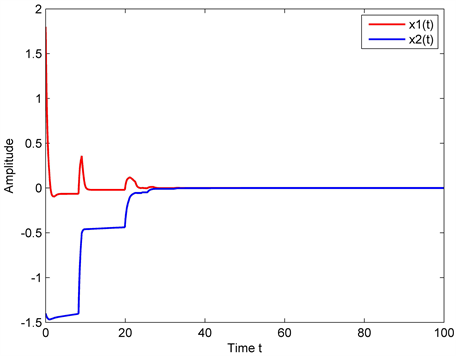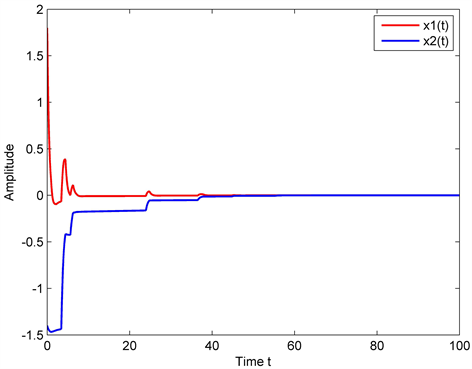# 一类中立型Markovian跳跃系统的渐近稳定性Asymptotic Stability of a Class of Neutral Markovian Jump Systems

DOI: 10.12677/AAM.2019.83060, PDF, 下载: 332  浏览: 443  科研立项经费支持

Abstract: Stability is one of the most important properties of dynamical system, which has important theo-retical significance to solve practical problems. The existence of time-delays is the root of system performance difference and systematic instability, so it has been considered by many scientists and scholars at home and abroad. In this paper, the asymptotic stability of a class of neutral Markovian jump systems is considered. Firstly, the Lyapunov function is constructed and the conditions for asymptotic stability are obtained by using Ito’s lemma and Jensen’s inequality. Secondly, the LMI toolbox in MATLAB is used to verify the correctness of the results. Finally, two examples are given to verify the validity of the method.

1. 引言

2. 问题描述

$\left\{\begin{array}{l}\stackrel{˙}{x}\left(t\right)-{C}_{\left({r}_{t},t\right)}\stackrel{˙}{x}\left(t-\tau \left(t\right)\right)={A}_{\left({r}_{t},t\right)}x\left(t\right)+{B}_{\left({r}_{t},t\right)}x\left(t-h\left(t\right)\right)+{D}_{\left({r}_{t},t\right)}{\int }_{t-r}^{t}x\left(s\right)\text{d}s\hfill \\ x\left({t}_{0}+\theta \right)=0,\forall \theta \in \left[\rho ,0\right].\hfill \end{array}$ (1)

$\begin{array}{l}0\le \tau \left(t\right)\le \tau ,0<\stackrel{˙}{\tau }\left(t\right)\le {\mu }_{1}<1,\\ 0\le {h}_{0}\le h\left(t\right)\le h,0<\stackrel{˙}{h}\left(t\right)\le {\mu }_{2}<1.\end{array}$ (2)

${A}_{\left({r}_{t},t\right)},{B}_{\left({r}_{t},t\right)},{C}_{\left({r}_{t},t\right)}$${D}_{\left({r}_{t},t\right)}\in {\Re }^{n×n}$ 是已知含有状态转移概率的常数矩阵， $\left\{{r}_{t}\right\},t>0$ 在马尔科夫过程有限状态概率空间中 $\xi =\left\{1,2,3,\cdots ,N\right\}$ 取值，且有 $\Lambda =\left({\lambda }_{ij}\right)\left(i,j\in \xi \right)$ ，即

$P\left({r}_{\left(t+\Delta \right)}=j|{r}_{\left(t\right)}=i\right)=\left\{\begin{array}{ll}{\lambda }_{ij}\Delta +o\left(\Delta \right),\hfill & i\ne j\hfill \\ 1+{\lambda }_{ii}\Delta +o\left(\Delta \right),\hfill & i=j\hfill \end{array}$ (3)

$\Lambda =\left[\begin{array}{cccc}{\lambda }_{11}& ?& {\lambda }_{13}& {\lambda }_{14}\\ ?& {\lambda }_{22}& ?& ?\\ {\lambda }_{31}& ?& {\lambda }_{33}& {\lambda }_{34}\\ {\lambda }_{41}& ?& ?& ?\end{array}\right],$ (4)

${U}_{k}^{i}:=\left\{j:对于\text{ }j\in \xi ,\text{\hspace{0.17em}}{\lambda }_{ij}\text{ }已知\right\},$

${U}_{uk}^{i}:=\left\{j:对于\text{ }j\in \xi ,\text{\hspace{0.17em}}{\lambda }_{ij}\text{ }已知\right\}.$

3. 定义及引理

${\int }_{t=0}^{\infty }E\left\{{‖x\left(t,{x}_{0}\right)‖}^{\delta }\right\}\text{d}t<\infty$ (5)

$LV\left({x}_{t},t,i\right)=\underset{\Delta \to {0}^{+}}{\mathrm{lim}}\frac{1}{\Delta }\left[E\left\{V\left({x}_{t+\Delta },t+\Delta ,{r}_{t+\Delta }\right)|{x}_{t},{r}_{t}=i\right\}-V\left({x}_{t},t,i\right)\right]<0$ (6)

$-h{\int }_{t-h}^{t}{\stackrel{˙}{x}}^{\text{T}}\left(s\right)W\stackrel{˙}{x}\left(s\right)\text{d}s\le {\left[\begin{array}{c}x\left(t\right)\\ x\left(t-h\right)\end{array}\right]}^{\text{T}}\left[\begin{array}{cc}-W& W\\ W& -W\end{array}\right]\left[\begin{array}{c}x\left(t\right)\\ x\left(t-h\right)\end{array}\right]$ (7)

$\left(\alpha -\beta \right){\int }_{\beta }^{\alpha }{x}^{\text{T}}\left(s\right)Sx\left(s\right)\text{d}s\ge \left({\int }_{\beta }^{\alpha }{x}^{\text{T}}\left(s\right)\text{d}s\right)S\left({\int }_{\beta }^{\alpha }x\left(s\right)\text{d}s\right)$ (8)

4. 主要结果

$\Phi =\left[\begin{array}{ccccccc}{\varphi }_{11}& {\varphi }_{12}& {\varphi }_{13}& {\varphi }_{14}& W& {\varphi }_{16}& {\varphi }_{17}\\ \ast & {\varphi }_{22}& {\varphi }_{23}& {\varphi }_{24}& 0& 0& {\varphi }_{27}\\ \ast & \ast & {\varphi }_{33}& {\varphi }_{34}& 0& {\varphi }_{36}& 0\\ \ast & \ast & \ast & {\varphi }_{44}& 0& 0& {\varphi }_{47}\\ \ast & \ast & \ast & \ast & -W& 0& 0\\ \ast & \ast & \ast & \ast & \ast & {\varphi }_{66}& 0\\ \ast & \ast & \ast & \ast & \ast & \ast & {\varphi }_{77}\end{array}\right]<0$ (8)

$\begin{array}{l}\underset{j={U}_{k}^{i}}{\sum }{\lambda }_{ij}\left({Q}_{j}-{Q}_{i}\right)<0;\underset{j={U}_{k}^{i}}{\sum }{\lambda }_{ij}\left({R}_{j}-{R}_{i}\right)<0,\\ {P}_{j}-{P}_{i}\le 0,j\in {U}_{uk}^{i},j\ne i;{Q}_{j}-{Q}_{i}\le 0,j\in {U}_{uk}^{i},j\ne i;\\ {Q}_{j}-{Q}_{i}\ge 0,j\in {U}_{k}^{i},j=i;{R}_{j}-{R}_{i}\le 0,j\in {U}_{uk}^{i},j\ne i;\\ {R}_{j}-{R}_{i}\ge 0,j\in {U}_{k}^{i},j=i.\end{array}$

${\varphi }_{11}={P}_{i}{A}_{i}+{A}_{i}^{\text{T}}{P}_{i}+{Q}_{i}+h{S}_{1}-\frac{1-{\mu }_{2}}{h}{S}_{3}-W-\left(\frac{{h}_{0}^{4}}{4}+{h}_{0}^{2}\right)T+rO+{M}_{1}{A}_{i}+\underset{j=1}{\overset{N}{\sum }}{\lambda }_{ij}\left({P}_{j}-{P}_{i}\right)$ ,

${\varphi }_{12}=h{S}_{2}-{M}_{1}+{A}_{i}^{\text{T}}{M}_{2}$ , ${\varphi }_{13}={P}_{i}{B}_{i}+\frac{1-{\mu }_{2}}{h}{S}_{3}+{M}_{1}{B}_{i}$ ,

${\varphi }_{14}={P}_{i}{C}_{i}+{M}_{1}{C}_{i}+{A}_{i}^{\text{T}}{M}_{3}$ , ${\varphi }_{16}=-\frac{1-{\mu }_{2}}{h}{S}_{2}^{\text{T}}+{h}_{0}^{2}T$ ,

${\varphi }_{17}={P}_{i}{D}_{i}+{M}_{1}{D}_{i}$ , ${\varphi }_{22}={R}_{i}+{\tau }^{2}W-{M}_{2}$ , ${\varphi }_{23}={B}_{i}^{\text{T}}{M}_{2}$ ,

${\varphi }_{24}={C}_{i}^{\text{T}}{M}_{2}-{M}_{3}$ , ${\varphi }_{27}={D}_{i}^{\text{T}}{M}_{2}$ , ${\varphi }_{33}=-\left(1-{\mu }_{2}\right){Q}_{i}$ ,

${\varphi }_{34}={B}_{i}^{\text{T}}{M}_{3}$ , ${\varphi }_{36}=\frac{1-{\mu }_{2}}{h}{S}_{2}^{\text{T}}$ , ${\varphi }_{44}=-\left(1-{\mu }_{1}\right){R}_{i}+2{M}_{3}{C}_{i}$ ,

${\varphi }_{47}={M}_{3}{D}_{i}$ , ${\varphi }_{66}=-\frac{1-{\mu }_{2}}{h}{S}_{1}$ , ${\varphi }_{77}=-\frac{1}{r}O$ .

$V\left({x}_{t},t,{r}_{t}\right)=\underset{n=1}{\overset{7}{\sum }}{V}_{n}\left({x}_{t},t,{r}_{t}\right)$ (9)

${V}_{1}\left({x}_{t},t,{r}_{t}\right)={x}^{\text{T}}\left(t\right){P}_{i}x\left(t\right)$

${V}_{2}\left({x}_{t},t,{r}_{t}\right)={\int }_{t-h\left(t\right)}^{t}{x}^{\text{T}}\left(s\right){Q}_{i}x\left(s\right)\text{d}s$

${V}_{3}\left({x}_{t},t,{r}_{t}\right)={\int }_{t-\tau \left(t\right)}^{t}{\stackrel{˙}{x}}^{\text{T}}\left(s\right){R}_{i}\stackrel{˙}{x}\left(s\right)\text{d}s$

${V}_{4}\left({x}_{t},t,{r}_{t}\right)={\int }_{t-h\left(t\right)}^{t}\left(h\left(t\right)-t+s\right){\xi }^{\text{T}}\left(s\right)S\xi \left(s\right)\text{d}s$

${V}_{5}\left({x}_{t},t,{r}_{t}\right)={\int }_{-\tau \left(t\right)}^{t}{\int }_{t+\theta }^{t}{\stackrel{˙}{x}}^{\text{T}}\left(s\right)\tau \left(t\right)W\stackrel{˙}{x}\left(s\right)\text{d}s\text{d}\theta$

${V}_{6}\left({x}_{t},t,{r}_{t}\right)=\frac{{h}_{0}{}^{2}}{2}{\int }_{-{h}_{0}}^{0}{\int }_{\theta }^{0}{\int }_{t+\lambda }^{t}{x}^{\text{T}}\left(s\right)Tx\left(s\right)\text{d}s\text{d}\theta \text{d}\lambda$

${V}_{7}\left({x}_{t},t,{r}_{t}\right)={\int }_{-r}^{t}{\int }_{t+\theta }^{t}{x}^{\text{T}}\left(s\right)Ox\left(s\right)\text{d}s\text{d}\theta$

$\begin{array}{c}L{V}_{1}\left({x}_{t},t,i\right)=2{x}^{\text{T}}\left(t\right){P}_{i}\stackrel{˙}{x}\left(t\right)+{x}^{\text{T}}\left(t\right)\underset{j=1}{\overset{N}{\sum }}{\lambda }_{ij}{P}_{j}x\left(t\right)\\ =2{x}^{\text{T}}\left(t\right){P}_{i}\left[{A}_{i}x\left(t\right)+{B}_{i}x\left(t-h\left(t\right)\right)+{C}_{i}\stackrel{˙}{x}\left(t-\tau \left(t\right)\right)+{D}_{i}{\int }_{t-r}^{t}x\left(s\right)\text{d}s\right]+{x}^{\text{T}}\left(t\right)\underset{j=1}{\overset{N}{\sum }}{\lambda }_{ij}{P}_{j}x\left(t\right)\\ ={x}^{\text{T}}\left(t\right)\left[{P}_{i}{A}_{i}+{A}_{i}^{\text{T}}{P}_{i}\right]x\left(t\right)+2{x}^{\text{T}}\left(t\right){P}_{i}{B}_{i}x\left(t-h\left(t\right)\right)+2{x}^{\text{T}}\left(t\right){P}_{i}{C}_{i}\stackrel{˙}{x}\left(t-\tau \left(t\right)\right)\\ \text{\hspace{0.17em}}\text{\hspace{0.17em}}+2{x}^{\text{T}}\left(t\right){P}_{i}{D}_{i}{\int }_{t-r}^{t}x\left(s\right)\text{d}s+{x}^{\text{T}}\left(t\right)\underset{j=1}{\overset{N}{\sum }}{\lambda }_{ij}{P}_{j}x\left(t\right)\end{array}$ (10)

$\begin{array}{c}L{V}_{2}\left({x}_{t},t,i\right)={x}^{\text{T}}\left(t\right){Q}_{i}x\left(t\right)-\left(1-\stackrel{˙}{h}\left(t\right)\right){x}^{\text{T}}\left(t-h\left(t\right)\right){Q}_{i}x\left(t-h\left(t\right)\right)+{\int }_{t-h\left(t\right)}^{t}{x}^{\text{T}}\left(s\right)\left(\underset{j=1}{\overset{N}{\sum }}{\lambda }_{ij}{Q}_{j}\right)x\left(s\right)\text{d}s\\ \le {x}^{\text{T}}\left(t\right){Q}_{i}x\left(t\right)-\left(1-{\mu }_{2}\right){x}^{\text{T}}\left(t-h\left(t\right)\right){Q}_{i}x\left(t-h\left(t\right)\right)+{\int }_{t-h\left(t\right)}^{t}{x}^{\text{T}}\left(s\right)\left(\underset{j=1}{\overset{N}{\sum }}{\lambda }_{ij}{Q}_{j}\right)x\left(s\right)\text{d}s\end{array}$ (11)

$\begin{array}{c}L{V}_{3}\left({x}_{t},t,i\right)={\stackrel{˙}{x}}^{\text{T}}\left(t\right){R}_{i}\stackrel{˙}{x}\left(t\right)-\left(1-\stackrel{˙}{\tau }\left(t\right)\right){\stackrel{˙}{x}}^{\text{T}}\left(t-\tau \left(t\right)\right){R}_{i}\stackrel{˙}{x}\left(t-\tau \left(t\right)\right)+{\int }_{t-\tau \left(t\right)}^{t}{x}^{\text{T}}\left(s\right)\left(\underset{j=1}{\overset{N}{\sum }}{\lambda }_{ij}{R}_{j}\right)x\left(s\right)\text{d}s\\ \le {\stackrel{˙}{x}}^{\text{T}}\left(t\right){R}_{i}\stackrel{˙}{x}\left(t\right)-\left(1-{\mu }_{1}\right){\stackrel{˙}{x}}^{\text{T}}\left(t-\tau \left(t\right)\right){R}_{i}\stackrel{˙}{x}\left(t-\tau \left(t\right)\right)+{\int }_{t-\tau \left(t\right)}^{t}{x}^{\text{T}}\left(s\right)\left(\underset{j=1}{\overset{N}{\sum }}{\lambda }_{ij}{R}_{j}\right)x\left(s\right)\text{d}s\end{array}$ (12)

$\begin{array}{c}L{V}_{4}\left({x}_{t},t,i\right)=h\left(t\right){\xi }^{\text{T}}\left(t\right)S\xi \left(t\right)-\left(1-\stackrel{˙}{h}\left(t\right)\right){\int }_{t-h\left(t\right)}^{t}{\xi }^{\text{T}}\left(s\right)S\xi \left(s\right)\text{d}s\\ \le h{\left[\begin{array}{c}x\left(t\right)\\ \stackrel{˙}{x}\left(t\right)\end{array}\right]}^{\text{T}}\left[\begin{array}{cc}{S}_{1}& {S}_{2}\\ {S}_{2}^{\text{T}}& {S}_{3}\end{array}\right]\left[\begin{array}{c}x\left(t\right)\\ \stackrel{˙}{x}\left(t\right)\end{array}\right]\\ \text{\hspace{0.17em}}\text{\hspace{0.17em}}-\frac{1-{\mu }_{2}}{h}{\left[{\int }_{t-h\left(t\right)}^{t}\left(\begin{array}{c}x\left(s\right)\\ \stackrel{˙}{x}\left(s\right)\end{array}\right)\text{d}s\right]}^{\text{T}}\left[\begin{array}{cc}{S}_{1}& {S}_{2}\\ {S}_{2}^{\text{T}}& {S}_{3}\end{array}\right]\left[{\int }_{t-h\left(t\right)}^{t}\left(\begin{array}{c}x\left(s\right)\\ \stackrel{˙}{x}\left(s\right)\end{array}\right)\text{d}s\right]\\ \le h{\left[\begin{array}{c}x\left(t\right)\\ \stackrel{˙}{x}\left(t\right)\end{array}\right]}^{\text{T}}\left[\begin{array}{cc}{S}_{1}& {S}_{2}\\ {S}_{2}^{\text{T}}& {S}_{3}\end{array}\right]\left[\begin{array}{c}x\left(t\right)\\ \stackrel{˙}{x}\left(t\right)\end{array}\right]\\ \text{\hspace{0.17em}}\text{\hspace{0.17em}}-\frac{1-{\mu }_{2}}{h}{\left[\begin{array}{c}{\int }_{t-h\left(t\right)}^{t}x\left(s\right)\text{d}s\\ x\left(t\right)-x\left(t-h\left(t\right)\right)\end{array}\right]}^{\text{T}}\left[\begin{array}{cc}{S}_{1}& {S}_{2}\\ {S}_{2}^{\text{T}}& {S}_{3}\end{array}\right]\left[\begin{array}{c}{\int }_{t-h\left(t\right)}^{t}x\left(s\right)\text{d}s\\ x\left(t\right)-x\left(t-h\left(t\right)\right)\end{array}\right]\end{array}$

$\begin{array}{c}L{V}_{4}\left({x}_{t},t,i\right)\le x{\left(t\right)}^{\text{T}}\left(h{S}_{1}-\frac{1-{\mu }_{2}}{h}{S}_{3}\right)x\left(t\right)+{\stackrel{˙}{x}}^{\text{T}}\left(t\right)h{S}_{2}^{\text{T}}x\left(t\right)+x{\left(t\right)}^{\text{T}}h{S}_{2}\stackrel{˙}{x}\left(t\right)+{\stackrel{˙}{x}}^{\text{T}}\left(t\right)h{S}_{3}\stackrel{˙}{x}\left(t\right)\\ \text{\hspace{0.17em}}\text{\hspace{0.17em}}\text{ }-\left({\int }_{t-h\left(t\right)}^{t}{x}^{\text{T}}\left(s\right)\text{d}s\right)\left(\frac{1-{\mu }_{2}}{h}{S}_{1}\right)\left({\int }_{t-h\left(t\right)}^{t}x\left(s\right)\text{d}s\right)-x{\left(t\right)}^{\text{T}}\left(\frac{1-{\mu }_{2}}{h}{S}_{2}^{\text{T}}\right)\left({\int }_{t-h\left(t\right)}^{t}x\left(s\right)\text{d}s\right)\\ \text{\hspace{0.17em}}\text{\hspace{0.17em}}\text{ }+{x}^{\text{T}}\left(t-h\left(t\right)\right)\left(\frac{1-{\mu }_{2}}{h}{S}_{2}^{\text{T}}\right)\left({\int }_{t-h\left(t\right)}^{t}x\left(s\right)\text{d}s\right)-\left({\int }_{t-h\left(t\right)}^{t}{x}^{\text{T}}\left(s\right)\text{d}s\right)\left(\frac{1-{\mu }_{2}}{h}{S}_{2}\right)x\left(t\right)\\ \text{\hspace{0.17em}}\text{\hspace{0.17em}}\text{ }+\left({\int }_{t-h\left(t\right)}^{t}{x}^{\text{T}}\left(s\right)\text{d}s\right)\left(\frac{1-{\mu }_{2}}{h}{S}_{2}\right)x\left(t-h\left(t\right)\right)+{x}^{\text{T}}\left(t\right)\left(\frac{1-{\mu }_{2}}{h}{S}_{3}\right)x\left(t-h\left(t\right)\right)\\ \text{\hspace{0.17em}}\text{\hspace{0.17em}}\text{ }+{x}^{\text{T}}\left(t-h\left(t\right)\right)\left(\frac{1-{\mu }_{2}}{h}{S}_{3}\right)x\left(t\right)-{x}^{\text{T}}\left(t-h\left(t\right)\right)\left(\frac{1-{\mu }_{2}}{h}{S}_{3}\right)x\left(t-h\left(t\right)\right)\end{array}$ (14)

$L{V}_{5}\left({x}_{t},t,i\right)={\tau }^{2}\left(t\right){\stackrel{˙}{x}}^{\text{T}}\left(t\right)W\stackrel{˙}{x}\left(t\right)-{\int }_{t-\tau \left(t\right)}^{t}{\stackrel{˙}{x}}^{\text{T}}\left(s\right)\tau W\stackrel{˙}{x}\left(s\right)\text{d}s$

$\begin{array}{c}L{V}_{5}\left({x}_{t},t,i\right)\le {\tau }^{2}{\stackrel{˙}{x}}^{\text{T}}\left(t\right)W\stackrel{˙}{x}\left(t\right)+\left[\begin{array}{cc}{x}^{\text{T}}\left(t\right)& {x}^{\text{T}}\left(t-\tau \left(t\right)\right)\end{array}\right]\left[\begin{array}{cc}-W& W\\ W& -W\end{array}\right]\left[\begin{array}{c}x\left(t\right)\\ x\left(t-\tau \left(t\right)\right)\end{array}\right]\\ ={\tau }^{2}{\stackrel{˙}{x}}^{\text{T}}\left(t\right)W\stackrel{˙}{x}\left(t\right)+{x}^{\text{T}}\left(t\right)\left(-W\right)x\left(t\right)+{x}^{\text{T}}\left(t-\tau \left(t\right)\right)Wx\left(t\right)\\ \text{\hspace{0.17em}}\text{\hspace{0.17em}}+{x}^{\text{T}}\left(t\right)Wx\left(t-\tau \left(t\right)\right)+{x}^{\text{T}}\left(t-\tau \left(t\right)\right)\left(-W\right)x\left(t-\tau \left(t\right)\right)\end{array}$ (15)

$\begin{array}{c}L{V}_{6}\left({x}_{t},t,i\right)=\frac{{\left({h}_{0}^{2}\right)}^{2}}{4}{x}^{\text{T}}\left(t\right)Tx\left(t\right)-\frac{{h}_{0}^{2}}{2}{\int }_{-{\tau }_{0}}^{0}{\int }_{t+\theta }^{t}{x}^{\text{T}}\left(s\right)Tx\left(s\right)\text{d}s\text{d}\theta \\ \le \frac{{h}_{0}^{4}}{4}{x}^{\text{T}}\left(t\right)Tx\left(t\right)-\left[{h}_{0}{x}^{\text{T}}\left(t\right)-\left({\int }_{t-h\left(t\right)}^{t}{x}^{\text{T}}\left(s\right)\text{d}s\right)T\left({h}_{0}x\left(t\right)-{\int }_{t-h\left(t\right)}^{t}x\left(s\right)\text{d}s\right)\right]\\ \le {x}^{\text{T}}\left(t\right)\left(\frac{{h}_{0}^{4}}{4}T-{h}_{0}^{2}T\right)x\left(t\right)+{x}^{\text{T}}\left(t\right)\left({h}_{0}^{2}T\right)\left({\int }_{t-h\left(t\right)}^{t}x\left(s\right)\text{d}s\right)\\ \text{\hspace{0.17em}}\text{\hspace{0.17em}}+\left({\int }_{t-h\left(t\right)}^{t}{x}^{\text{T}}\left(s\right)\text{d}s\right)\left({h}_{0}^{2}T\right)x\left(t\right)-\left({\int }_{t-h\left(t\right)}^{t}{x}^{\text{T}}\left(s\right)\text{d}s\right)T\left({\int }_{t-h\left(t\right)}^{t}x\left(s\right)\text{d}s\right)\end{array}$ (16)(17)

$\begin{array}{c}0=\left[\begin{array}{ccc}{x}^{\text{T}}\left(t\right)& {\stackrel{˙}{x}}^{\text{T}}\left(t\right)& {\stackrel{˙}{x}}^{\text{T}}\left(t-\tau \left(t\right)\right)\end{array}\right]\left[\begin{array}{c}{M}_{1}\\ {M}_{2}\\ {M}_{3}\end{array}\right]\\ \text{\hspace{0.17em}}\text{\hspace{0.17em}}×\left[-\stackrel{˙}{x}\left(t\right)+{A}_{\left({r}_{t},t\right)}x\left(t\right)+{B}_{\left({r}_{t},t\right)}x\left(t-h\left(t\right)\right)+{C}_{\left({r}_{t},t\right)}\stackrel{˙}{x}\left(t-\tau \left(t\right)\right)+{D}_{\left({r}_{t},t\right)}{\int }_{t-r}^{t}x\left(s\right)\text{d}s\right]\end{array}$ (18)

$-{x}^{\text{T}}\left(t\right)\left(\underset{j=1}{\overset{N}{\sum }}{\lambda }_{ij}{P}_{i}\right)x\left(t\right)=0,\forall i\in \xi$ (19)

$-{\int }_{t-h\left(t\right)}^{t}{x}^{\text{T}}\left(s\right)\left(\underset{j=1}{\overset{N}{\sum }}{\lambda }_{ij}{Q}_{i}\right)x\left(t\right)\text{d}s=0,\forall i\in \xi$ (20)

$-{\int }_{t-\tau \left(t\right)}^{t}{x}^{\text{T}}\left(s\right)\left(\underset{j=1}{\overset{N}{\sum }}{\lambda }_{ij}{R}_{i}\right)x\left(t\right)\text{d}s=0,\forall i\in \xi$ (21)

$\begin{array}{c}LV\left({x}_{t},t,i\right)=\underset{n=1}{\overset{7}{\sum }}L{V}_{n}\left({x}_{t},t,i\right)\\ \le {\Psi }^{\text{T}}\left(t\right)\Phi \Psi \left(t\right)+{x}^{\text{T}}\left(t\right)\underset{j\in {U}_{uk}^{i}}{\sum }{\lambda }_{ij}\left({P}_{j}-{P}_{i}\right)x\left(s\right)+{\int }_{t-h\left(t\right)}^{t}{x}^{\text{T}}\left(s\right)\underset{j\in {U}_{k}^{i}}{\sum }{\lambda }_{ij}\left({Q}_{j}-{Q}_{i}\right)x\left(s\right)\text{d}s\\ \text{\hspace{0.17em}}\text{\hspace{0.17em}}+{\int }_{t-h\left(t\right)}^{t}{x}^{\text{T}}\left(s\right)\underset{j\in {U}_{uk}^{i}}{\sum }{\lambda }_{ij}\left({Q}_{j}-{Q}_{i}\right)x\left(s\right)\text{d}s+{\int }_{t-\tau \left(t\right)}^{t}{x}^{\text{T}}\left(s\right)\underset{j\in {U}_{k}^{i}}{\sum }{\lambda }_{ij}\left({R}_{j}-{R}_{i}\right)x\left(s\right)\text{d}s\\ \text{\hspace{0.17em}}\text{\hspace{0.17em}}+{\int }_{t-\tau \left(t\right)}^{t}{x}^{\text{T}}\left(s\right)\underset{j\in {U}_{uk}^{i}}{\sum }{\lambda }_{ij}\left({R}_{j}-{R}_{i}\right)x\left(s\right)\text{d}s\end{array}$

${\Psi }^{\text{T}}\left(t\right)=\left[\begin{array}{cccc}{x}^{\text{T}}\left(t\right)& {\stackrel{˙}{x}}^{\text{T}}\left(t\right)& {x}^{\text{T}}\left(t-h\left(t\right)\right)& {\stackrel{˙}{x}}^{\text{T}}\left(t-\tau \left(t\right)\right)\end{array}\text{}\begin{array}{ccc}{x}^{\text{T}}\left(t-\tau \left(t\right)\right)& {\int }_{t-h\left(t\right)}^{t}{x}^{\text{T}}\left(s\right)\text{d}s& {\int }_{t-r}^{t}{x}^{\text{T}}\left(s\right)\text{d}s\end{array}\right]$

${\int }_{t=0}^{\infty }E\left\{{‖x\left(t,{x}_{0}\right)‖}^{\delta }\right\}\text{d}t<\infty$ (22)

$\left\{\begin{array}{l}\stackrel{˙}{x}\left(t\right)-{C}_{\left({r}_{t},t\right)}\stackrel{˙}{x}\left(t-\tau \left(t\right)\right)={A}_{\left({r}_{t},t\right)}x\left(t\right)+{B}_{\left({r}_{t},t\right)}x\left(t-h\left(t\right)\right)+{D}_{\left({r}_{t},t\right)}{\int }_{t-r\left(t\right)}^{t}x\left(s\right)\text{d}s,\hfill \\ x\left({t}_{0}+\theta \right)=0,\forall \theta \in \left[0,\rho \right].\hfill \end{array}$ (23)

$\stackrel{˜}{\Phi }=\left[\begin{array}{ccccccc}{\stackrel{˜}{\varphi }}_{11}& {\stackrel{˜}{\varphi }}_{12}& {\stackrel{˜}{\varphi }}_{13}& {\stackrel{˜}{\varphi }}_{14}& W& {\stackrel{˜}{\varphi }}_{16}& {\stackrel{˜}{\varphi }}_{17}\\ \ast & {\stackrel{˜}{\varphi }}_{22}& {\stackrel{˜}{\varphi }}_{23}& {\stackrel{˜}{\varphi }}_{24}& 0& 0& {\stackrel{˜}{\varphi }}_{27}\\ \ast & \ast & {\stackrel{˜}{\varphi }}_{33}& {\stackrel{˜}{\varphi }}_{34}& 0& {\stackrel{˜}{\varphi }}_{36}& 0\\ \ast & \ast & \ast & {\stackrel{˜}{\varphi }}_{44}& 0& 0& {\stackrel{˜}{\varphi }}_{47}\\ \ast & \ast & \ast & \ast & -W& 0& 0\\ \ast & \ast & \ast & \ast & \ast & {\stackrel{˜}{\varphi }}_{66}& 0\\ \ast & \ast & \ast & \ast & \ast & \ast & {\stackrel{˜}{\varphi }}_{77}\end{array}\right]<0$ (24)

$\begin{array}{l}\underset{j={U}_{k}^{i}}{\sum }{\lambda }_{ij}\left({Q}_{j}-{Q}_{i}\right)<0;\underset{j={U}_{k}^{i}}{\sum }{\lambda }_{ij}\left({R}_{j}-{R}_{i}\right)<0,\\ {P}_{j}-{P}_{i}\le 0,j\in {U}_{uk}^{i},j\ne i;{Q}_{j}-{Q}_{i}\le 0,j\in {U}_{uk}^{i},j\ne i;\\ {Q}_{j}-{Q}_{i}\ge 0,j\in {U}_{k}^{i},j=i;{R}_{j}-{R}_{i}\le 0,j\in {U}_{uk}^{i},j\ne i;\\ {R}_{j}-{R}_{i}\ge 0,j\in {U}_{k}^{i},j=i.\end{array}$

${\stackrel{˜}{\varphi }}_{11}={P}_{i}{A}_{i}+{A}_{i}^{\text{T}}{P}_{i}+{Q}_{i}+h{S}_{1}-\frac{1-{\mu }_{2}}{h}{S}_{3}-W-\left(\frac{{h}_{0}^{4}}{4}+{h}_{0}^{2}\right)T+rO+{M}_{1}{A}_{i}+\underset{j=1}{\overset{N}{\sum }}{\lambda }_{ij}\left({P}_{j}-{P}_{i}\right)$

${\stackrel{˜}{\varphi }}_{12}=h{S}_{2}-{M}_{1}+{A}_{i}^{\text{T}}{M}_{2}$ , ${\stackrel{˜}{\varphi }}_{13}={P}_{i}{B}_{i}+\frac{1-{\mu }_{2}}{h}{S}_{3}+{M}_{1}{B}_{i}$ , ${\stackrel{˜}{\varphi }}_{14}={P}_{i}{C}_{i}+{M}_{1}{C}_{i}+{A}_{i}^{\text{T}}{M}_{3}$ ,

${\stackrel{˜}{\varphi }}_{16}=-\frac{1-{\mu }_{2}}{h}{S}_{2}^{\text{T}}+{h}_{0}^{2}T$ , ${\stackrel{˜}{\varphi }}_{17}={P}_{i}{D}_{i}+{M}_{1}{D}_{i}$ , ${\stackrel{˜}{\varphi }}_{22}={R}_{i}+{\tau }^{2}W-{M}_{2}$ , ${\stackrel{˜}{\varphi }}_{23}={B}_{i}^{\text{T}}{M}_{2}$ , ${\stackrel{˜}{\varphi }}_{24}={C}_{i}^{\text{T}}{M}_{2}-{M}_{3}$ , ${\stackrel{˜}{\varphi }}_{27}={D}_{i}^{\text{T}}{M}_{2}$ , ${\stackrel{˜}{\varphi }}_{33}=-\left(1-{\mu }_{2}\right)Q$ , ${\stackrel{˜}{\varphi }}_{34}={B}_{i}^{\text{T}}{M}_{3}$ , ${\stackrel{˜}{\varphi }}_{36}=\frac{1-{\mu }_{2}}{h}{S}_{2}^{\text{T}}$ , ${\stackrel{˜}{\varphi }}_{44}=-\left(1-{\mu }_{1}\right)R+2{M}_{3}{C}_{i}$ , ${\stackrel{˜}{\varphi }}_{47}={M}_{3}{D}_{i}$ , ${\stackrel{˜}{\varphi }}_{66}=-\frac{1-{\mu }_{2}}{h}{S}_{1}$ , ${\stackrel{˜}{\varphi }}_{77}=-\frac{1-{\mu }_{3}}{r}O$ .

$0\le r\left(t\right)\le \tau ,0<\stackrel{˙}{r}\left(t\right)\le {\mu }_{3}<1.$

$\begin{array}{c}\stackrel{˜}{L}{V}_{7}\left({x}_{t},t,{r}_{t}\right)=r\left(t\right){x}^{\text{T}}\left(t\right)Ox\left(t\right)-{\int }_{t-r\left(t\right)}^{t}{x}^{\text{T}}\left(s\right)Ox\left(s\right)\text{d}s\\ =r\left(t\right){x}^{\text{T}}\left(t\right)Ox\left(t\right)-\frac{1-\stackrel{˙}{r}\left(t\right)}{r\left(t\right)}\left({\int }_{t-r\left(t\right)}^{t}{x}^{\text{T}}\left(s\right)\text{d}s\right)O\left({\int }_{t-r\left(t\right)}^{t}x\left(s\right)\text{d}s\right)\\ \le r{x}^{\text{T}}\left(t\right)Ox\left(t\right)-\frac{1-{\mu }_{3}}{r}\left({\int }_{t-r\left(t\right)}^{t}{x}^{\text{T}}\left(s\right)\text{d}s\right)O\left({\int }_{t-r\left(t\right)}^{t}x\left(s\right)\text{d}s\right)\end{array}$ (25)

5. 仿真算例

$\left\{\begin{array}{l}\stackrel{˙}{x}\left(t\right)-{C}_{\left({r}_{t},t\right)}\stackrel{˙}{x}\left(t-\tau \left(t\right)\right)={A}_{\left({r}_{t},t\right)}x\left(t\right)+{B}_{\left({r}_{t},t\right)}x\left(t-h\left(t\right)\right)+{D}_{\left({r}_{t},t\right)}{\int }_{t-r}^{t}x\left(s\right)\text{d}s,\hfill \\ x\left({t}_{0}+\theta \right)=0,\forall \theta \in \left[\rho ,0\right].\hfill \end{array}$

${A}_{1}=\left[\begin{array}{cc}-1.2& 0.1\\ -0.1& -1\end{array}\right]$ , ${A}_{2}=\left[\begin{array}{cc}-1.2& 0.15\\ 0.1& -1.5\end{array}\right]$ , ${B}_{1}=\left[\begin{array}{cc}-0.6& 0.7\\ -1& -0.8\end{array}\right]$ , ${B}_{2}=\left[\begin{array}{cc}-0.15& 1.5\\ -1& -0.8\end{array}\right]$ ,

${C}_{1}={C}_{2}=\left[\begin{array}{cc}c& 0\\ 0& c\end{array}\right]$ ,  , ${D}_{2}=\left[\begin{array}{cc}0.15& -1\\ -1& 0\end{array}\right]$ ,

$h=0.1,\tau =0.1,{\mu }_{1}=0.75,{\mu }_{2}=0.5,r=0.75,{h}_{0}=0.1.$Figure 1. The state trajectory of a neutral Markovian jump system with time-varying delay and distributed delay

$\left\{\begin{array}{l}\stackrel{˙}{x}\left(t\right)-{C}_{\left({r}_{t},t\right)}\stackrel{˙}{x}\left(t-\tau \left(t\right)\right)={A}_{\left({r}_{t},t\right)}x\left(t\right)+{B}_{\left({r}_{t},t\right)}x\left(t-h\left(t\right)\right)+{D}_{\left({r}_{t},t\right)}{\int }_{t-r\left(t\right)}^{t}x\left(s\right)\text{d}s,\hfill \\ x\left({t}_{0}+\theta \right)=0,\forall \theta \in \left[0,\rho \right].\hfill \end{array}$

${A}_{1}=\left[\begin{array}{cc}-2& 1\\ 1& -0.9\end{array}\right]$ , ${B}_{1}=\left[\begin{array}{cc}-1& -0.2\\ 1& 2\end{array}\right]$ , ${C}_{1}=\left[\begin{array}{cc}-1& -2\\ -1& 2\end{array}\right]$ , ${D}_{1}=\left[\begin{array}{cc}-1& 0\\ 0& 1\end{array}\right]$ ,

${A}_{2}=\left[\begin{array}{cc}-1& 1.5\\ 0& 1\end{array}\right]$ , ${B}_{2}=\left[\begin{array}{cc}2.5& 0\\ 0& 1\end{array}\right]$ , ${C}_{2}=\left[\begin{array}{cc}0.15& 0\\ -1& 0\end{array}\right]$ , ${D}_{2}=\left[\begin{array}{cc}0.15& -1\\ -1& 0\end{array}\right]$ .

$h=0.1,\tau =0.1,{\mu }_{1}=0.75,{\mu }_{2}=0.5,r=0.75,{h}_{0}=0.1,{\mu }_{3}\text{=}0.75.$Figure 2. The state trajectory of a neutral Markov jumping system with time-varying delay and time-varying distributed delay

6. 总结

NOTES

*通讯作者。

  Krasovskii, K. and Lidskii, E. (1961) Analysis and Design of Controllers in Systems with Random Attributes. Automat Remote Control, 22, 1021-1025.  Amri, I., Soudani, D. and Benreje, M. (2009) Delay Dependent Robust Expo-nential Stability for Uncertain Systems with Time Varying Delays and Nonlinear Perturbations. The 10th International Conference on Sciences and Techniques of Automatic Control & Computer Engineering, Hammamet.  Amri, I. and Soudani, D. (2010) Robust Exponential Stability of Uncertain Perturbed Systems with Time Varying Delays. IFAC Proceedings Volumes, 43, 522-527. https://doi.org/10.3182/20100712-3-FR-2020.00085  Amri, I., Soudani, D. and Benrejeb, M. (2008) New Condition for Exponential Stability of Linear Time Delay Systems: LMI Approach. The 9th International Conference on Sciences and Techniques of Automatic Control and Computer Engineering STA’08, Sousse.  Li, X.G. and Zhu, X.J. (2008) Stability Analysis of Neutral Systems with Distributed Delays. Automatica, 44, 2197-2201. https://doi.org/10.1016/j.automatica.2007.12.009  Chen, Y., Xue, A.K., Lu, R.Q. and Zhou, S.S. (2008) On Robustly Exponential Stability of Uncertain Neutral Systems with Time-Varying Delays and Nonlinear Perturbations. Nonlinear Analysis, 68, 2464-2470. https://doi.org/10.1016/j.na.2007.01.070  He, Y., Wang, Q.G., Lin, C. and Wu, M. (2007) De-lay-Range-Dependent Stability for Systems with Time-Varying Delay. Automatica, 43, 371-376. https://doi.org/10.1016/j.automatica.2006.08.015  Kwon, O.M., Park, J.H. and Lee, S.M. (2010) An Improved Delay-Dependent Criterion for Asymptotic Stability of Uncertain Dynamic Systems with Time-Varying Delays. Journal of Optimization Theory and Applications, 145, 343-353.  Niamsup, P., Mukdasai, K. and Phat, V.N. (2008) Improved Exponential Stability for Time-Varying Systems with Nonlinear Delayed Perturbations. Applied Mathematics and Computation, 204, 490-495. https://doi.org/10.1016/j.amc.2008.07.022  Park, J.H. (2005) Novel Robust Stability Criterion for a Class of Neutral Systems with Mixed Delays and Nonlinear Perturbations. Applied Mathematics and Computation, 161, 413-421. https://doi.org/10.1016/j.amc.2003.12.036  Shen, H., Su, L. and Park, J.H. (2015) Further Results on Stochastic Admissibility for Singular Markov Jump Systems Using a Dissipative Constrained Condition. ISA Transactions, 59, 65-71. https://doi.org/10.1016/j.isatra.2015.10.001  Long, S., Zhong, S., Zhu, H., et al. (2014) Delay-Dependent Stochastic Admissibility for a Class of Discrete-Time Nonlinear Singular Markovian Jump Systems with Time-Varying Delay. Communications in Nonlinear Science & Numerical Simulation, 19, 673-685. https://doi.org/10.1016/j.cnsns.2013.07.014  Xiong, L.L., Zhong, S.M. and Li, D.Y. (2009) Novel De-lay-Dependent Asymptotical Stability of Neutral Systems with Nonlinear Perturbations. Journal of Computational and Applied Mathematics, 232, 505-513. https://doi.org/10.1016/j.cam.2009.06.026  Han, Q.L., et al. (2004) Robust Stability of Linear Neutral Systems with Nonlinear Parameter Perturbations. IEE Proceedings of Control Theory and Applications, 15, 539-546. https://doi.org/10.1049/ip-cta:20040785  Zhang, J.H., Shi, P. and Qiu, J.Q. (2008) Robust Stability Criteria for Uncertain Neutral System with Time Delay and Nonlinear Uncertainties. Chaos, Solutions and Fractals, 38, 160-167. https://doi.org/10.1016/j.chaos.2006.10.068  Mazhoud, I., Amri, I. and Soudani, D. (2015) Asymptotical Stability Criterion for a Class of Nonlinear Neutral Systems with Mixed Time-Varying Delays. IEEE 12th International Multi-Conference on Systems, Signals & Devices (SSD), Mahdia, 16-19 March 2015.  Zou, Z. and Wang, Y. (2006) New Stability Criterion for a Class of Linear Systems with Time-Varying Delay and Nonlinear Perturbations. IEE Proceedings of Control Theory and Applications, 153, 623-626. https://doi.org/10.1049/ip-cta:20045258  Han, Q.L. (2004) On Robust Stability for a Class of Linear Systems with Time-Delay and Nonlinear Perturbations. Computers & Mathematics with Applications, 23, 235-241.  Beyd, S., Ei, L., Ghaoui, I., Feron, E. and Balakrishnan, V. (1994) linear Matrix Lnequalities in Systems and Control Theory. Studies in Applied Mathematics, 105, 1-205.